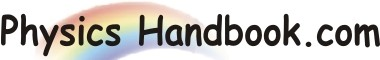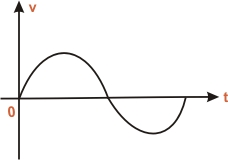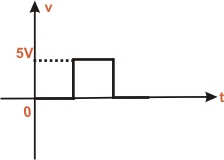HOME TOPICS DEFINITIONS TABLES LAWS INVENTIONS EXPERIMENTS QUIZ VIDEOS
 A B C D E F G H I J K L M N O P Q R S T U V W X Y Z
Analog and Digital Signal
(I) Analog signal : A continuously varying signal (voltage or current) is called an analog signal. For example, an alternating voltage varying sinusoidally is an analog signal see the fig (a). If such an analog signal is applied to the input of a transistor amplifier, the output voltage will also vary  sinusoidally. This is the analog operation i.e. the output voltage can have an infinite number of values. Due to many-valued output, the analog operation is less reliable.Fig (a)
(II) Digital Signal : A signal (voltage or current) that can have only two discrete value is called a digital signal. For example, a square wave is a digital signal see the fig(b). It is because this signal has two values viz. +5 V and 0 V and no other value. These values are labeled as High and Low. The High voltage is +5 V and the Low voltage is 0 V.Fig (b)
If proper digital signal is applied to the input of a transistor, the transistor can be driven between cut off and saturation. In other words, the transistor will have two-state operation i.e. output is either low or high. Since digital operation has only two states (i.e. ON or OFF), it is far more reliable than many-valued analog operation. It is because with two-state operation, all the signal are easily recognized as either low or high.